# Sample Data: Car Evaluation

Predicting car acceptability by attribute.

The Car Evaluation Database was created to test a model of hierarchical decision making. The model consists of three categories; price, technical features and comfort. Price is defined by purchase price, and maintenance costs. Technical features are defined by safety and comfort. Comfort can be further defined by the number doors, passenger capacity and cargo capacity. The goal was to predict the acceptability of a car based on these input attributes.

## Examples

### Basic Examples

Retrieve the resource:

 In:=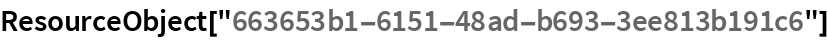Out=Retrieve the default content:

 In:=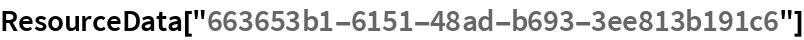Out=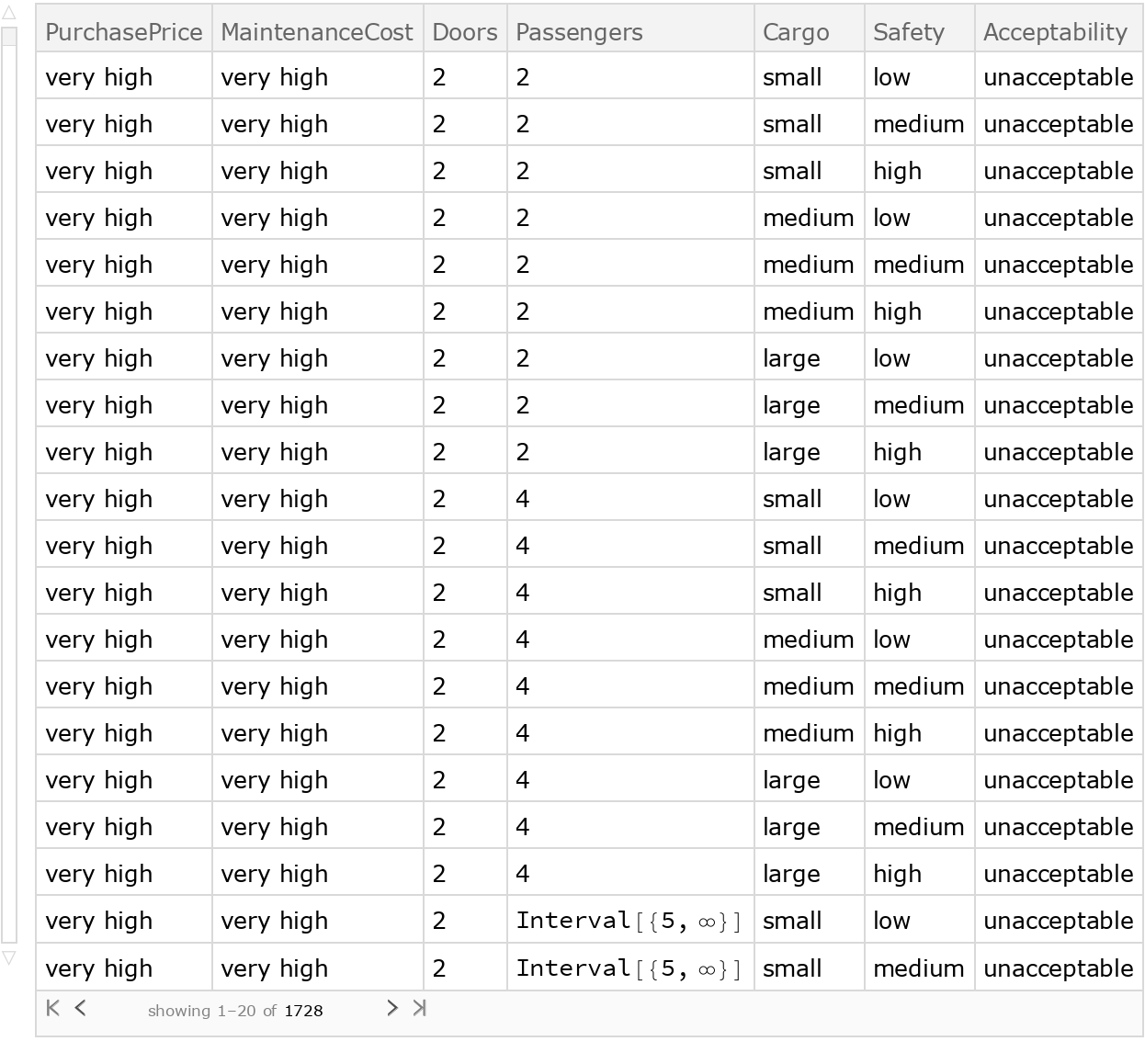### Analysis

Shuffle the data randomly:

 In:=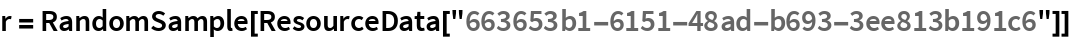Out=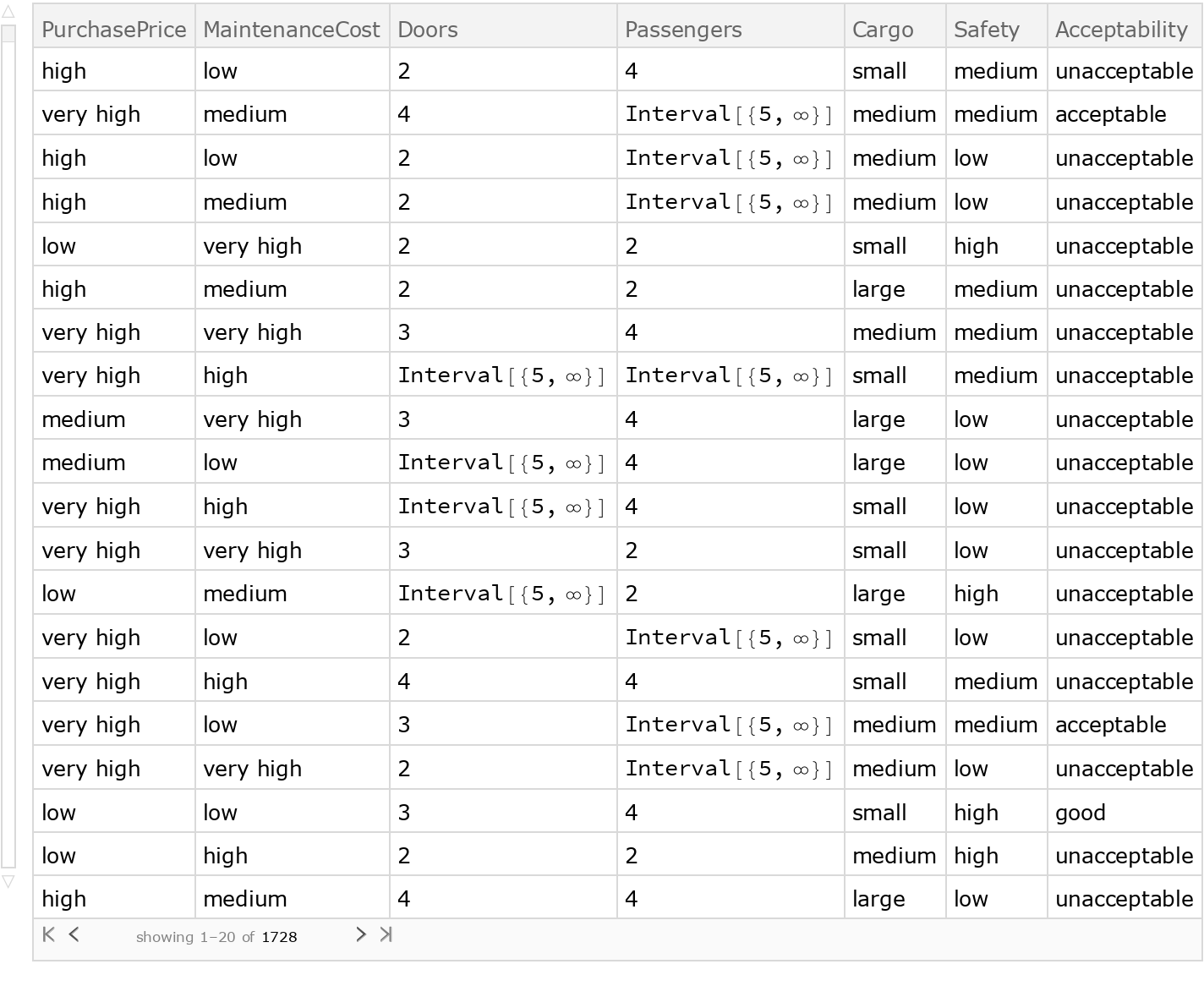Create a training dataset using 80% of the original dataset:

 In:=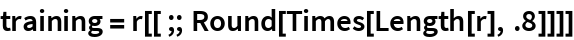Out=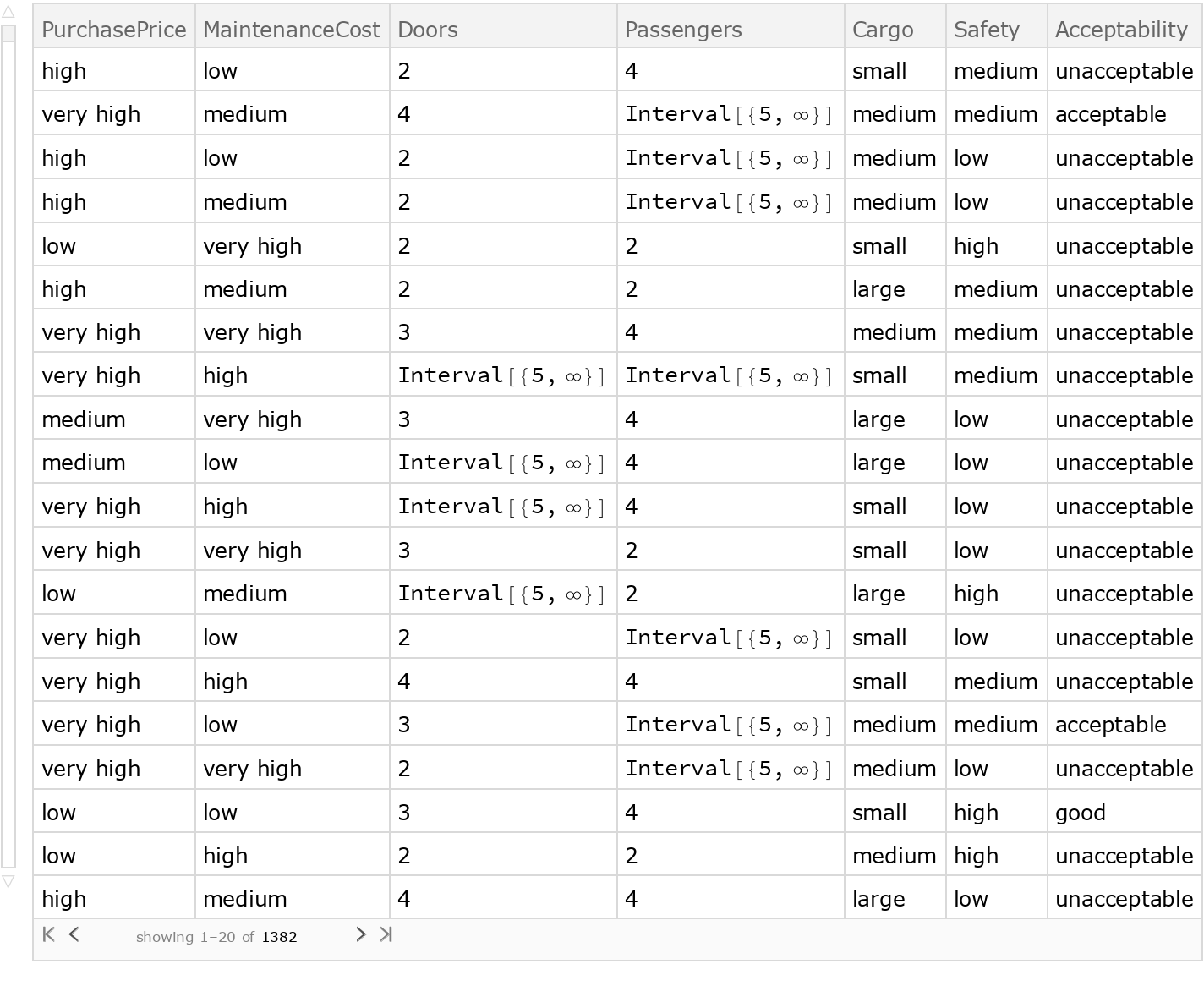Create a testing dataset using the remaining 20% of the original dataset:

 In:=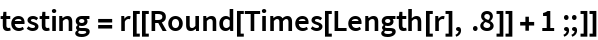Out=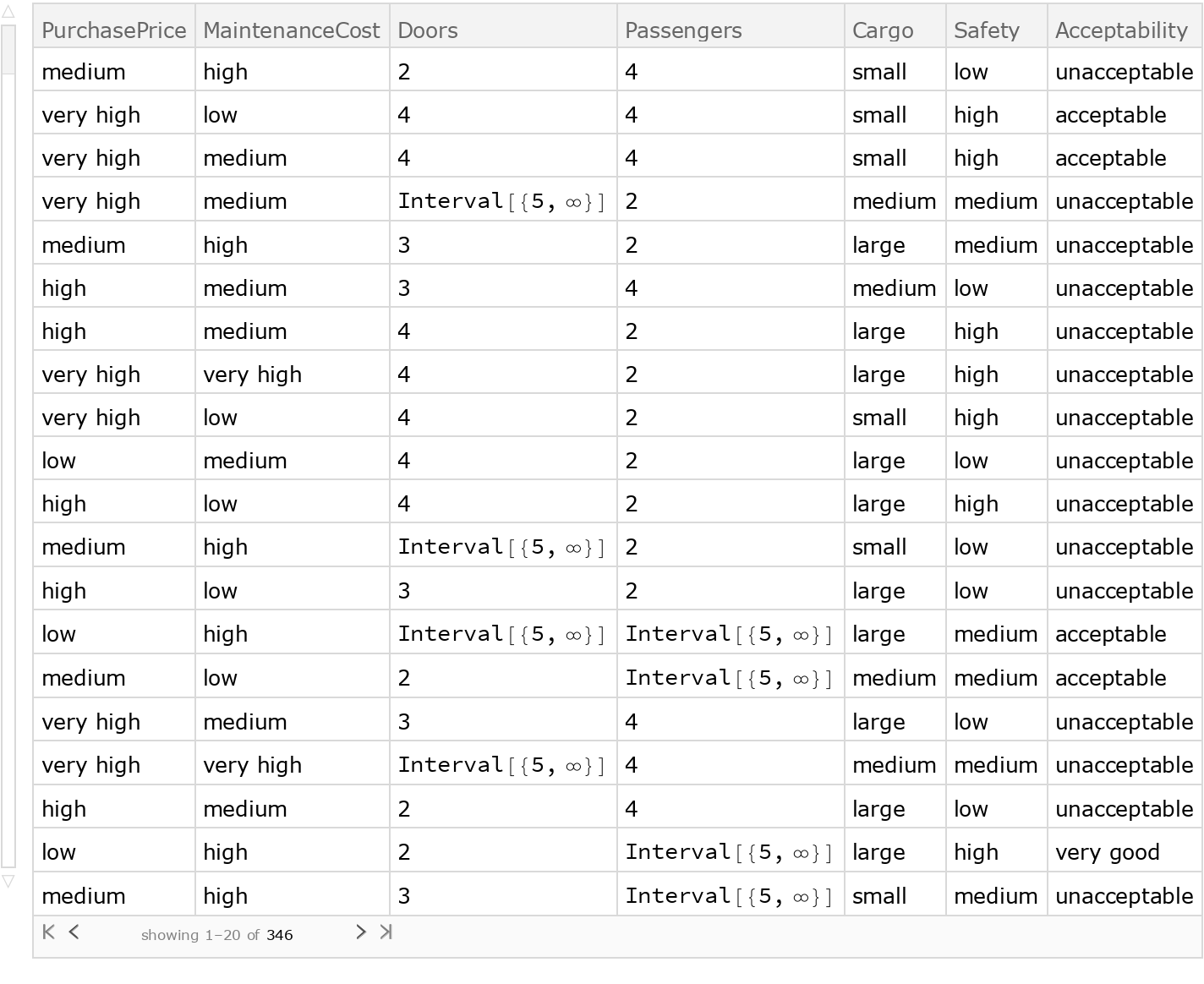Train a classifier:

 In:=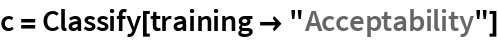Out=Obtain general information about the classifier:

 In:=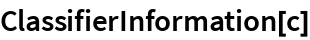Out=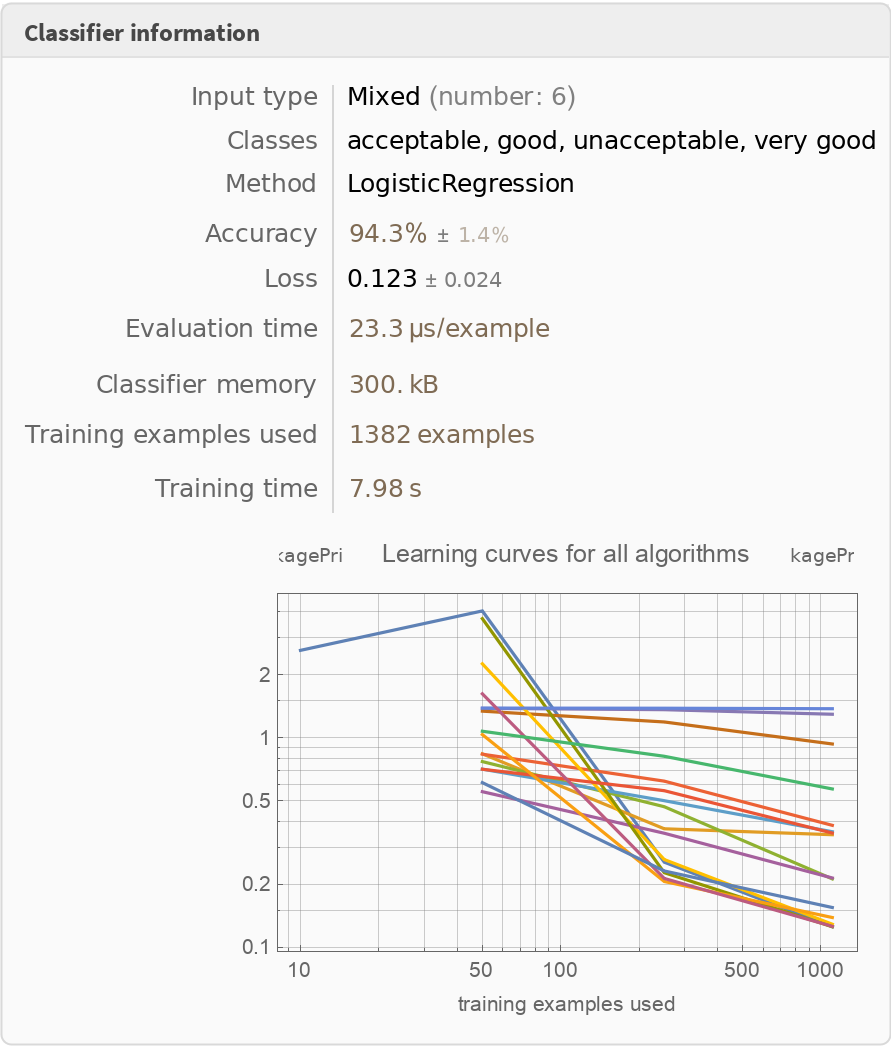Test the classifier:

 In:=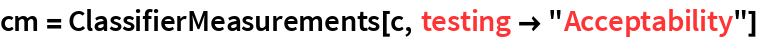Out=Visualize the accuracy of the classifier:

 In:=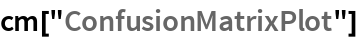Out=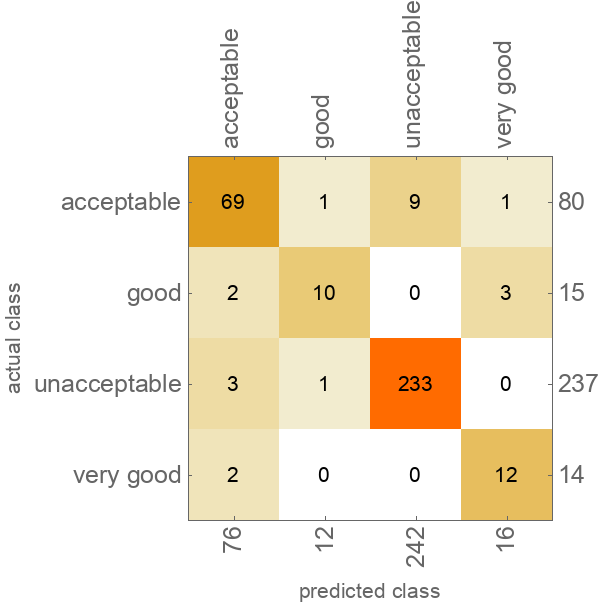Wolfram Research, "Sample Data: Car Evaluation" from the Wolfram Data Repository (2017)